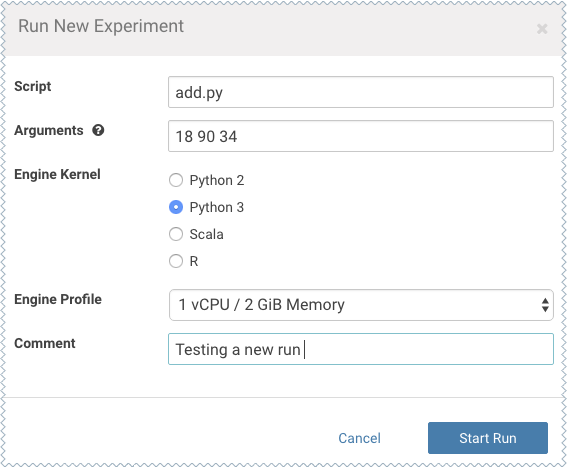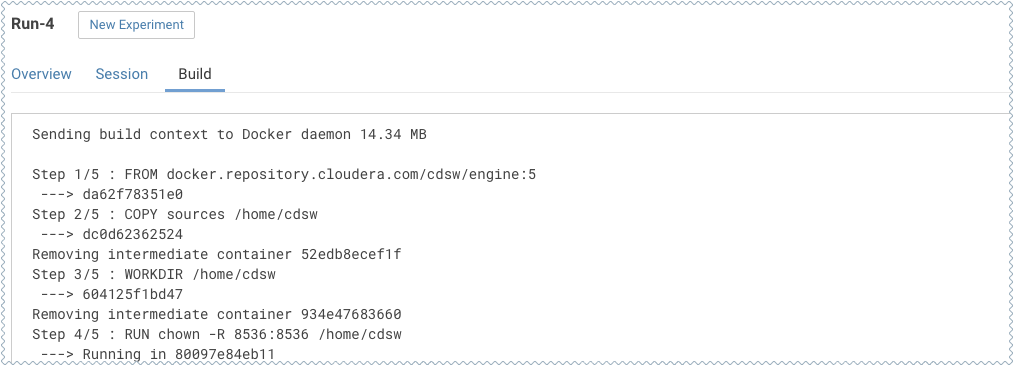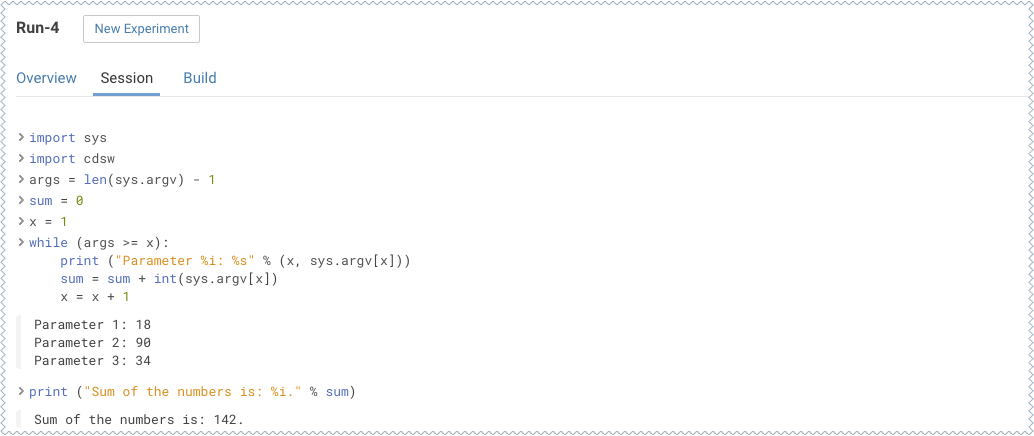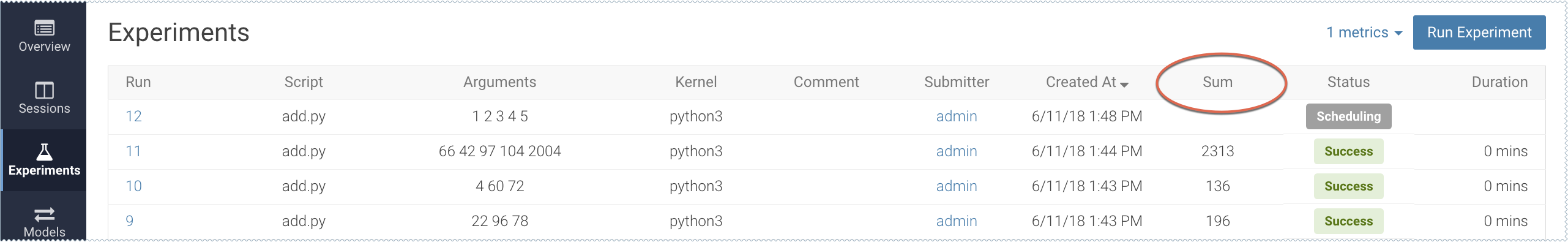# Running an Experiment (Quick Start)

The following steps describe how to launch an experiment from the Workbench console. In this example we are going to run a simple script that adds all the numbers passed as arguments to the experiment.

1. Go to the project Overview page.
2. Click Open Workbench.
3. Create/modify any project code as needed. You can also launch a session to simultaneously test code changes on the interactive console as you launch new experiments.
As an example, you can run this Python script that accepts a series of numbers as command-line arguments and prints their sum.
``````import sys
import cdsw

args = len(sys.argv) - 1
sum = 0
x = 1

while (args >= x):
print ("Argument %i: %s" % (x, sys.argv[x]))
sum = sum + int(sys.argv[x])
x = x + 1

print ("Sum of the numbers is: %i." % sum)``````
4. To test the script, launch a Python session and run the following command from the workbench command prompt:
``!python add.py 1 2 3 4``
5. Click Run Experiment. If you're already in an active session, click Run > Run Experiment. Fill out the following fields:
• Script - Select the file that will be executed for this experiment.

• Arguments - If your script requires any command line arguments, enter them here.
• Engine Kernel and Resource Profile - Select the kernel and computing resources needed for this experiment.

For this example we will run the `add.py` script and pass some numbers as arguments.6. Click Start Run.
7. To track progress for the run, go back to the project Overview. On the left navigation bar click Experiments. You should see the experiment you've just run at the top of the list. Click on the Run ID to view an overview for each individual run. Then click Build.
On this Build tab you can see realtime progress as Cloudera Data Science Workbench builds the Docker image for this experiment. This allows you to debug any errors that might occur during the build stage.8. Once the Docker image is ready, the run will begin execution. You can track progress for this stage by going to the Session tab.
For example, the Session pane output from running `add.py` is:9. (Optional) The `cdsw` library that is bundled with Cloudera Data Science Workbench includes some built-in functions that you can use to compare experiments and save any files from your experiments.
For example, to track the sum for each run, add the following line to the end of the `add.py` script.
``cdsw.track_metric("Sum", sum)``
This will be tracked in the Experiments table: